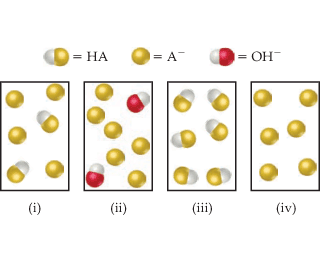# Problem: The following drawings represent solutions at various stages of the titration of a weak acid, HA, with NaOH. (The Na+ ions and water molecules have been omitted for clarity.) To which of the following regions of the titration curve does each drawing correspond.(iii)

###### FREE Expert Solution

We are asked which of the following regions of the titration curve does each drawing correspond

• The solution should be mostly HA.

before addition of NaOH: image (iii)

2) after addition of NaOH but before equivalence point

• HA should be lessened and A- should start to appear

after addition of NaOH but before equivalence point: image (i)

87% (301 ratings)###### Problem Details

The following drawings represent solutions at various stages of the titration of a weak acid, HA, with NaOH. (The Na+ ions and water molecules have been omitted for clarity.)To which of the following regions of the titration curve does each drawing correspond.

(iii)